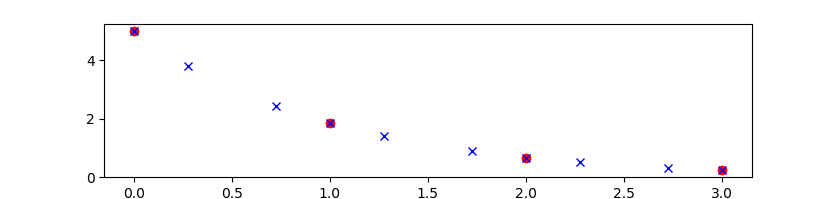## APM.NODES - APMonitor OptionGlobal Options |Local Options
 Type: Integer, Input
Default Value: 3
Allowable Range: 2-6
Description: Nodes in each horizon step


NODES are the number of collocation points in the span of each time segment. For dynamic problems, the time segments are linked together into a time horizon as shown in the figure below.In this example illustration, the number of nodes is 4 and the number of time segments is 3 such as defined by these 4 time points: [0,1,2,3]. Successive endpoints of the time segments are merged to form a chain of model predictions. The end designation can be used for the polynomial segments or the nodes with p(3)=p(end) and n(4)=n(end) for the example above. Increasing the number of nodes will generally improve the solution accuracy but also increase the problem size and computation time. Solution accuracy can also be improved by adding more time segments. APMonitor and APM Matlab use index-1 for the polynomial time positions while Gekko and Python use index-0. The node positions are index-1 for both APMonitor and Gekko. To facilitate common functions to fix or free initial or end points, the following Gekko functions are available.

from gekko import GEKKO
m = GEKKO()
x = m.Var()
m.fix_initial(x)
m.fix_final(x)
m.free_initial(x)
m.free_final(x)

A more detailed description of NODES is given in the lecture material on Orthogonal Collocation on Finite Elements with example problems. An additional example problem is shown below.

#### Example Problem

Solve the equation with NODES=4 at time points [0,1,2,3]:

$$\frac{dy}{dt}=-y$$

with initial condition y_0=5. Show the internal node solutions.

Solutionfrom gekko import GEKKO
m = GEKKO(remote=False) # create GEKKO model
y = m.Var(5.0,name='y') # create GEKKO variable
m.Equation(y.dt()==-y)  # create GEKKO equation
m.time = [0,1,2,3]      # time points
m.options.IMODE = 4     # simulation mode
m.options.NODES = 4     # set NODES=4
m.options.CSV_WRITE=2   # write results_all.json
#   with internal nodes
m.solve()               # solve
m.open_folder()         # open run folder to see
#   source and results files

import matplotlib.pyplot as plt
# plot at [0,1,2,3]
plt.plot(m.time,y,'ro')

# retrieve internal nodes from results_all.json
import json
with open(m.path+'//results_all.json') as f: